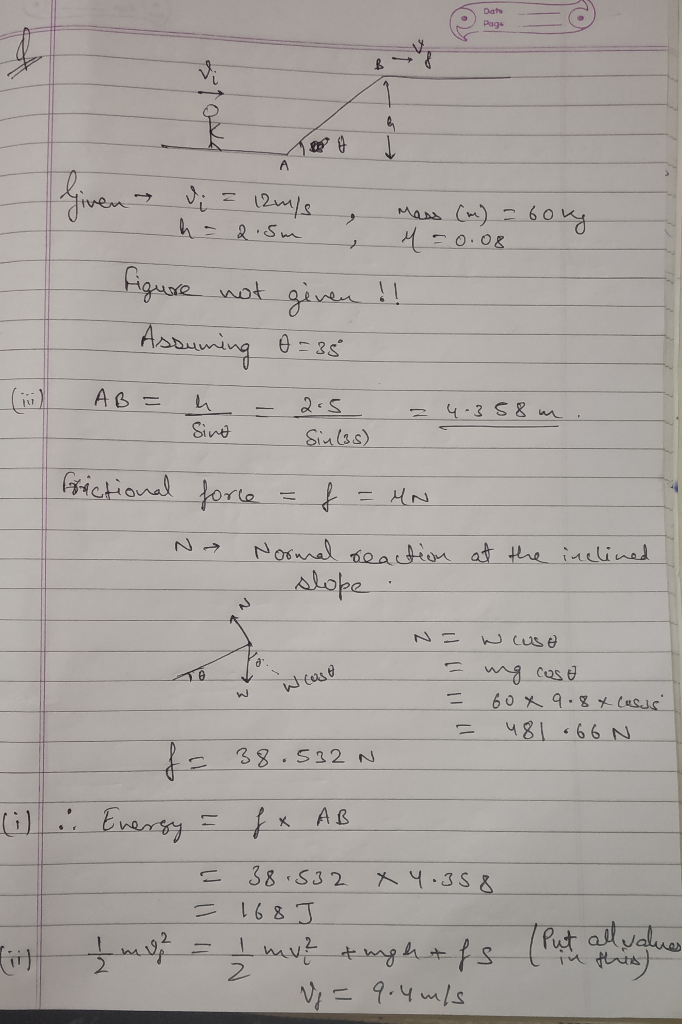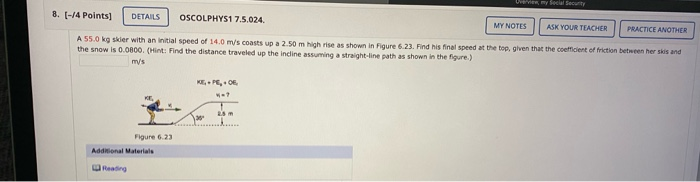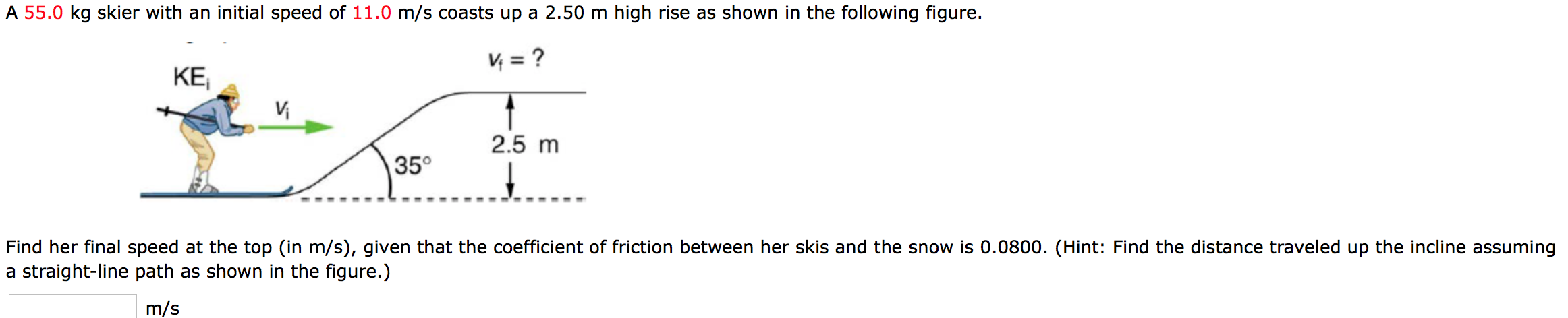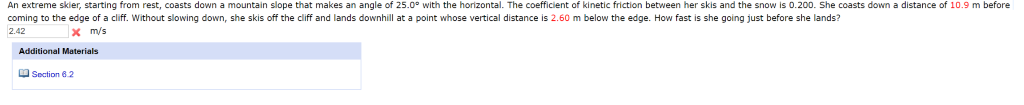Question

# A 60.0-kg skier with an initial speed of 12.0 m/s coasts up a 2.50-m high rise...

A 60.0-kg skier with an initial speed of 12.0 m/s coasts up a 2.50-m high rise as shown. You can assume she starts exactly at the bottom of the rise, and the coefficient of friction between her skis and all surfaces is 0.80. (a) Find the work done by friction during the climb. (b) Find her final speed at the top. (c) Find the distance she travels along the top horizontal surface before she comes to rest.#### Earn Coins

Coins can be redeemed for fabulous gifts.

Similar Homework Help Questions
• ### ​A 55.0 kg skier with an initial speed of 14.0 m/s coasts up a 2.50 m high rise as shown in Figure 6.23A 55.0 kg skier with an initial speed of 14.0 m/s coasts up a 2.50 m high rise as shown in Figure 6.23. Find his final speed at the top, given that the coefficient of friction between her skis and the snow is 0.0800. (Hint: Find the distance traveled up the incline assuming a straight-line path as shown in the figure.)

• ### A 55.0 kg skier with an initial speed of 11.0 m/s coasts up a 2.50 m...A 55.0 kg skier with an initial speed of 11.0 m/s coasts up a 2.50 m high rise as shown in the following figure. Vi = ? KE 2.5 m / 35° Find her final speed at the top (in m/s), given that the coefficient of friction between her skis and the snow is 0.0800. (Hint: Find the distance traveled up the incline assuming a straight-line path as shown in the figure.) m/s

• ### A 63.0–kg skier with an initial speed of 12.2 m/s coasts up a 33.0° incline to...

A 63.0–kg skier with an initial speed of 12.2 m/s coasts up a 33.0° incline to a rise of 2.64–m as shown in figure below. Find her final speed at the top, given that the coefficient of friction between her skis and the snow is 0.0760. (Hint: Find the distance traveled up the incline assuming a straight-line path as shown in the figure.) The skier’s initial kinetic energy is partially used in coasting to the top of a rise.

• ### A skier, starting from rest, coasts down a slope that makes a 25.0o angle with the...

A skier, starting from rest, coasts down a slope that makes a 25.0o angle with the horizontal. The coefficient of kinetic friction between the snow and the skis is 0.200. The skier travels along the slope a distance of 11.4 m before skiing off the edge of a cliff. The skier falls a vertical distance of 3.80 m before landing on another downhill slope. Determine the speed with which the skier lands.

• ### A skier with mass 64.0 kg starts at rest at the top of an 842 m...

A skier with mass 64.0 kg starts at rest at the top of an 842 m long ski slope, which makes an angle 13.0 ∘ with the horizontal. A typical coefficient of friction between skis and snow is 5.20×10−2. skiers don't go straight down the hill- they zigzag back and forth. Even though they still end up at the bottom of the hill, they've lost more energy to friction because friction is a non-conservative force. Let's say due to zigzagging,...

• ### A skier starting from rest coasts down a mountain that makes an angle of 25 degrees...

A skier starting from rest coasts down a mountain that makes an angle of 25 degrees with the horizontal. The coefficient of kinetic friction between her skis and the snow is 0.200. She coasts for a distance of 10.4 m before coming to the edge of a cliff. Without slowing down, she skis off the cliff and lands dwonhill at a point whose vertical distance is 3.50 m below the edge. How fast is she going before she lands? Please...

• ### An extreme skier, starting from rest, coasts down a mountain slope that makes an angle of...An extreme skier, starting from rest, coasts down a mountain slope that makes an angle of 25.0° with the horizontal. The coefficient of kinetic friction between her skis and the snow is 0.200. She coasts down a distance of 10.9 m before coming the edge of a cliff. Without slowing down, she skis off the cliff and lands downhill at a point whose vertical distance is 2.60 m below the edge. How fast is she going just before she lands?...

• ### a skier starts......

A skier starts from rest at the top of a hill that is inclined at 9.5° with the horizontal. The hillside is 155 m long, and the coefficient of friction between snowand skis is 0.0750. At the bottom of the hill, the snow is level and the coefficient of friction is unchanged. How far does the skier glide along the horizontalportion of the snow before coming to rest?____ m

• ### A skier starts from rest at the top of a hill that is inclined at 10.0°...

A skier starts from rest at the top of a hill that is inclined at 10.0° with respect to the horizontal. The hillside is 250 m long, and the coefficient of friction between snow and skis is 0.0750. At the bottom of the hill, the snow is level and the coefficient of friction is unchanged. How far does the skier glide along the horizontal portion of the snow before coming to rest?

• ### A 72.5-kg skier coasts up a snow-covered hill that makes an angle of 22.9 ° with...

A 72.5-kg skier coasts up a snow-covered hill that makes an angle of 22.9 ° with the horizontal. The initial speed of the skier is 8.71 m/s. After coasting a distance of 1.19 m up the slope, the speed of the skier is 3.74 m/s. (a) Find the work done by the kinetic frictional force that acts on the skis. (b) What is the magnitude of the kinetic frictional force?